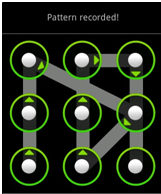DWITE
February 2013
Problem 5
Pattern Lock

A lot of phones nowadays are moving away from the standard number lock to using pattern locks. Given a 3 by 3 grid of dots, a pattern is defined to be a directed path amongst the 9 points of length at least 1 (Note: a path visits each point at most once). However, there's an extra condition: the path can't cross an unvisited vertex (i.e. you can't go from the top left dot to the bottom right dot unless the center dot has already been visited). For example, the following picture depicts one possible pattern for a 3 by 3 grid:One day you decide to find out exactly how secure this lock is by determining the number of possible patterns you can make for a 3 by 3 grid of up to a particular length.

Input Specifications
The first line of each test case contains a number N (1 <= N <= 8), representing the maximum length the pattern has to be.

Output Specifications
The output should contain the total possible number of patterns you can have for the 3 by 3 grid lock up to the given length.

Sample Input (only two test cases shown):
```
1
2
```
Sample Output (only two shown):
```
56
376
```